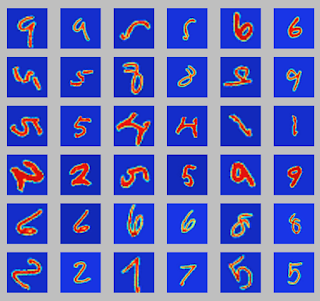## Posts

Showing posts from June, 2017

### End of the first work period

Hi all,

this is the end of this first period of GSoC! It was a challenging but very interesting period and I am very excited about the next two months. It is the first time where I have the opportunity to make something that has a real value, albeit small, on someone else's work. Even when I have to do small tasks, like structure the package or learn Tensorflow APIs, I do it with enthusiasm because I have very clear in mind the ultimate goal and the value that my efforts would bring. It may seem trivial, but for a student this is not the daily bread :) I really have fun coding for this project and I hope this will last until the end!

Speaking about the project, I've spent some time wondering which was the best solution to test the correct installation of the Tensorflow Python APIs on the machine. The last solution was putting the test in a function __nnet_init__ and calling it in the PKG_ADD (code in inst/__nnet_init__ in my repo ).

Regarding the code, in this last days I t…

### Train a Convolutional Neural Network for RegressionHello,

I spent the last period working mostly on Tensorflow, studying the APIs and writing some examples in order to explore the possible implementations of neural networks. For this goal, I chose an interesting example proposed in the Matlab examples at . The dataset is composed by 5000 images, rotated by an angle α, and a corresponding integer label (the rotation angle α). The goal is to make a regression to predict the angle of a rotated image and straighten it up.
All files can be found in tests/examples/cnn_linear_model in my repo .

I have kept the structure as in the Matlab example, but I generated a new dataset starting from LeCun's MNIST digits (datasets at ). Each image was rotated by a random angle between 0° and 70°, in order to keep the right orientation of the digits (code in dataset_generation.m). In Fig. 1 some rotated digits with the corresponding original digits.

The implemented linear model is: $\hat{Y} = \omega X + b$, where the weights $\omega$ and t…

### Package structureHello! During the first period of GSoC I have worked mostly on analyzing the Matlab structure of the net package in order to guarantee the compatibility throughout the whole project. The focus of the project is on the convolutional neural networks, about which I will write the next post.

Regarding the package structure, the core will be composed by three parts:

Layers:there are 11 types of layersthat I defined as Octave classes, using classdef. These layers can be concatenated in order to create a Layer object defining the architecture of the network. This will be the input for the training function. Training: the core of the project is the training function, which takes as input the data, the layers and some options and returns the network as output. Network:the network object has three methods (activations, classify and predict) that let the user compute the final classification and prediction.

I have already implemented a draft for the first point, the layers classes . Every l…